Function Repository Resource:

# TensorCoordinateTransform

Transform components of tensors with arbitrary rank with regard to their transformation behavior under any given mapping

Contributed by: Lars Ulke-Winter
 ResourceFunction["TensorCoordinateTransform"][tensor,mat] transform tensor components according to their transformation behavior matrix mat. All tensor slots are considered to be contravariant and not normalized.

## Details and Options

Generally tensor components (with mixed n×m-rank) transform from one system to another (ii') according to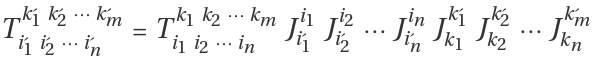.
The mapping from the old system to the new one is described in the matrix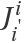for covariant transformation behavior (tensor components with lower indices) and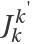for so-called contravariant tensor components (depicted with superscript indices).
Summation must be done for the same but opposing indices about the dimensions of the tensor coordinates. The fundamental relationship of the contrary transformation behavior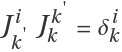ensures invariance of the tensor object T in all systems after contracting all opposing indices (e.g.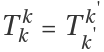). Due to this fact, a distinction of covariant and contravariant transformation behavior is not required in transformation relations with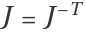, e.g. in transformations between orthonormal reference systems.
The input tensor can be of any rank and should be a List or an object of StructuredArray.
The resulting format is always a normal List.
Unlike TransformedField, the ResourceFunction["TensorCoordinateTransform"] result is not given with respect to a normalized basis by default. Using the option Normalize True forces a normalized basis.
The matrix mat defines the mapping between two coordinate systems (often their transposed Jacobian).
The following options are supported:
 "TransformationBehavior" "AllContravariant" transformation behavior of individual components "Normalize" False whether the components are represented with respect to a normalized basis
Values allowed by the option "TransformationBehavior" include "AllContravariant", "AllCovariant" and {"Con","Cov",}.

## Examples

### Basic Examples (1)

Apply a rotation transformation to a tensor:

 In:=Out=### Scope (13)

#### Tensor Components with Respect to Rotated Base Vectors (4)

Rotate vector components about the three axes counterclockwise:

 In:=Out=Change the axis:

 In:=Out=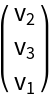Rotate the rank-2 tensor about the three axes counterclockwise:

 In:=Out=Rotate a rank-4 tensor with three symmetries about the three axes counterclockwise:

 In:=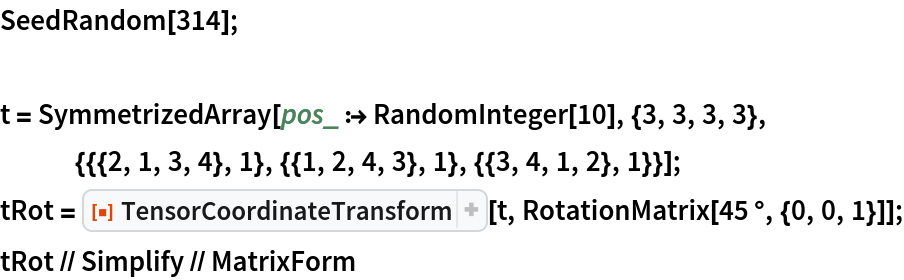Out=#### Transform Vector Components Regarding Another Affine Frame of Reference (2)

The contravariant vector components with respect to covariant bases are a=vjbj=vi'bi'. The old system is Cartesian (aj=vj):

 In:=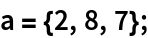Consider a transformation between new ei' and old ej covariant base vectors e1'=2e1+3e2+1e3, e2'=1e1+2e2+2e3 and e3'=1e1+2e3. Here are the contravariant components vi' of the new system:

 In:=Out=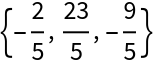They should be the same vector:

 In:=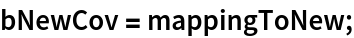In:=Out=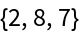In:=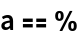Out=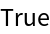The covariant vector components with respect to contravariant bases are a=vjbj=vi'bj'

 In:=Out=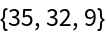Find related contravariant base vectors bi' by transforming the covariant base in contravariant sense (index juggling bi'bi'):

 In:=Out=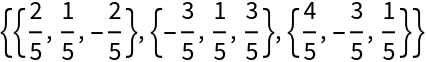In:=Out=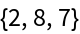In:=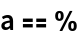Out=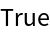Note that covariant and contravariant base vectors are inverse to each other bi'bj'=δi'j':

 In:=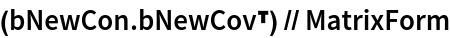Out=#### A Local Base on the Manifold of a Torus Surface (Curvilinear Coordinate System) (7)

Consider the aibicijklskltjbi=ci'j'k'l'sk'l'tj'bi'ai'bi'-invariant tensors in Cartesian frame:

 In:=The transformation from Cartesian to local torus coordinates (ii'):

 In:=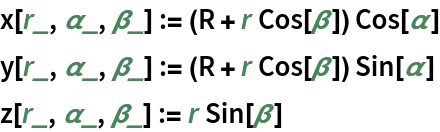The torus surface at α=β=02π, with R and r constant:

 In:=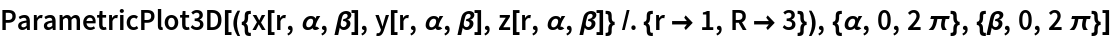Out=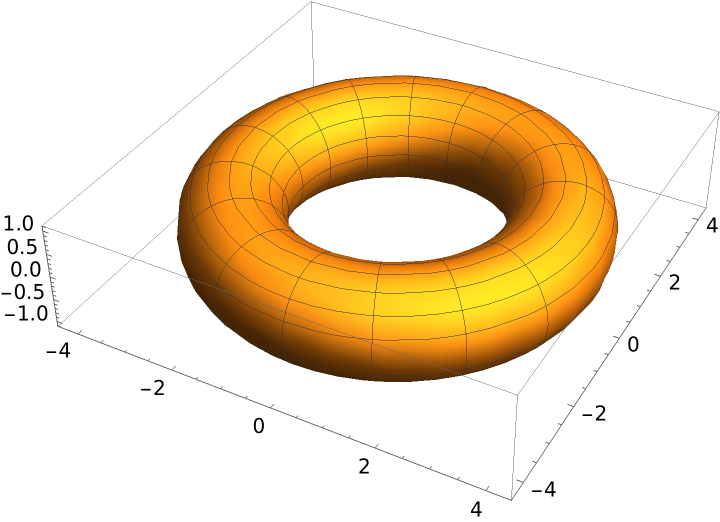Transform tensors' different ranks regarding the local torus base with respect to transformation behavior. This is faster than transformation of an entire assembled rank-7 tensor):

 In:=Local covariant base vector bi':

 In:=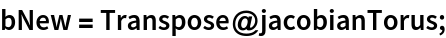Assemble the tensor term by contracting the right indices ci'j'k'l'sk'l'tj'biai'bi':

 In:=Out=In:=Out=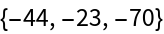The result should be the same in the Cartesian system:

 In:=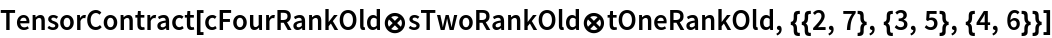Out=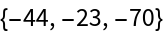### Options (7)

#### TransformationBehavior (2)

If the base vectors are not normalized ("Normalize"False), i.e. if they have local-dependent lengths (and are not orthogonal in general reference systems either), the corresponding tensor components are transformed according to their co- and contravariant transformation behaviors. This leads to different representations of the same tensor object.

Here is an example of all four transforming possibilities of a rank-2 tensor to a local, non-normalized cylindrical system:

 In:=All coordinates transform the contravariant tij (default):

 In:=Out=All coordinates transform the covariant tij:

 In:=Out=A tensor object with coordinates of mixed-transformation behavior tij:

 In:=Out=A tensor object with coordinates of opposite mixed-transformation behavior tji:

 In:=Out=Nomenclature referencing all indices together or each index separately is interchangeable:

 In:=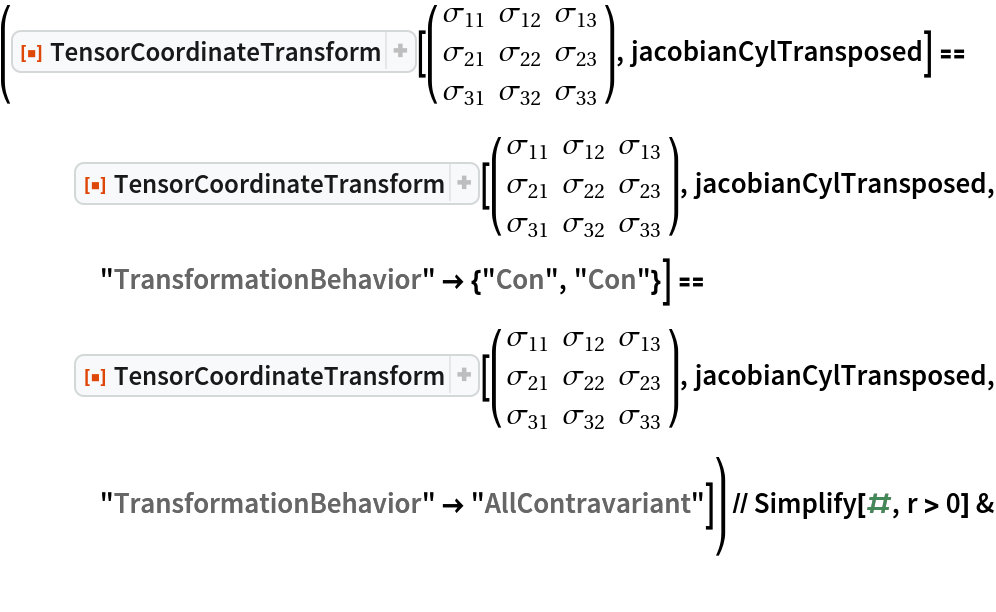Out=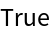This is analogous for the covariant transformation:

 In:=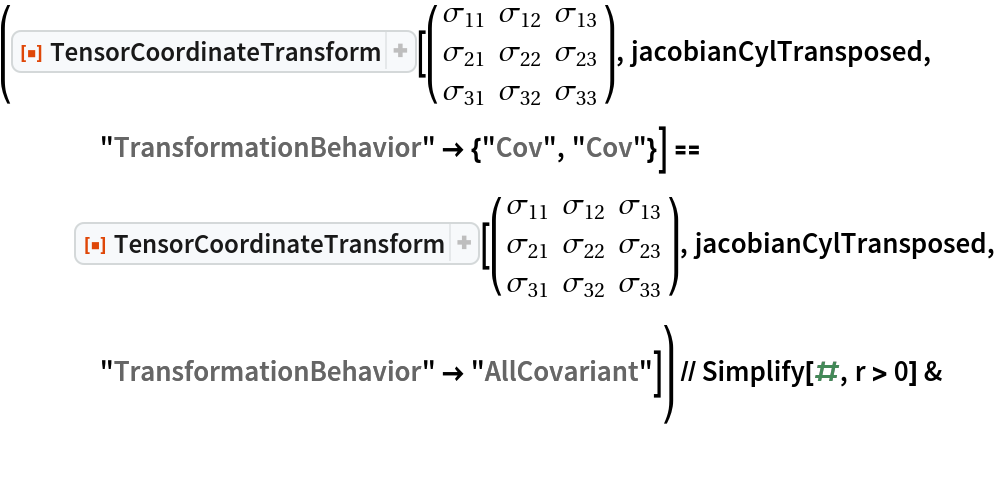Out=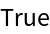#### Normalize (5)

Transformed contravariant vector components with respect to normalized base vectors:

 In:=Out=The same transformed contravariant vector components with respect to non-normalized base vectors (default):

 In:=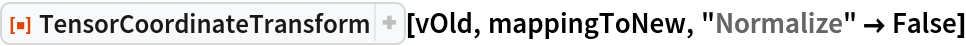Out=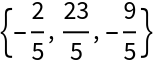Transformed covariant normalized base vectors:

 In:=Out=The contraction of the assigned tensors should be produce invariance:

 In:=Out=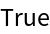Tensor components with respect to an orthonormal cylindrical system:

 In:=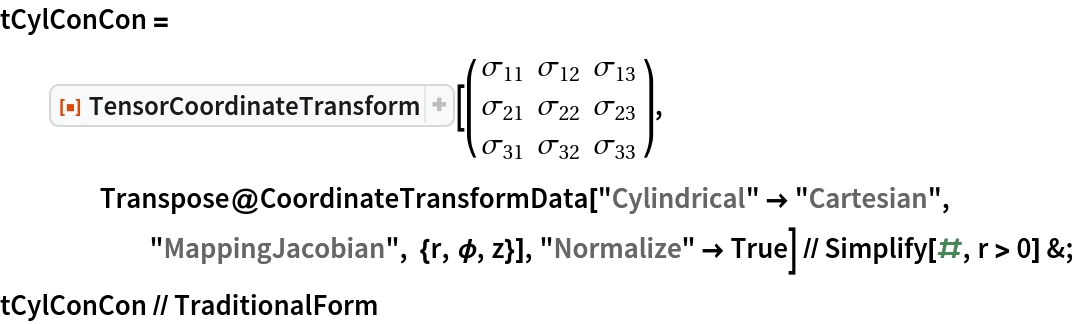Out=TransformedField also assumes a normalized base:

 In:=Out=In:=Out=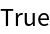With orthonormal reference systems (e.g. normalized cylindrical systems), no distinction between co- and contravariant transformation behavior is required:

 In:=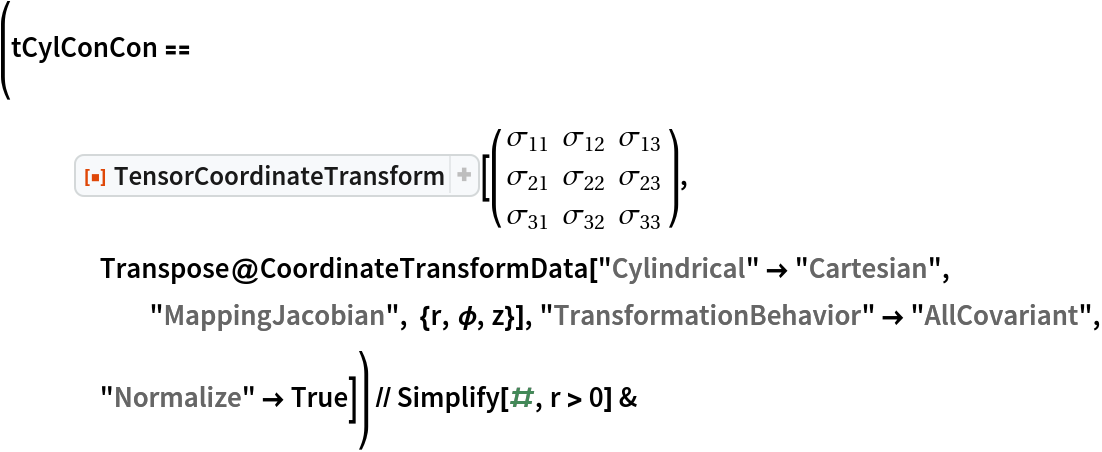Out=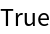### Applications (6)

#### Stiffness Components of the Rank-4 Elasticity Tensor with Different Material Symmetries (6)

 In:=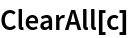Hook's general law describes a linear relationship between the components of the rank-2 stress tensor σ and the two-stage strain tensor ε using the rank-4 tensor C: σij=Cijklεkl with the symmetries σij=σji, εkl=εlk and Eijkl=Ejikl=Eijlk=Eklij.

Components of the general, fully anisotropic stiffness tensor with initial symmetries:

 In:=Out=The number of independent components:

 In:=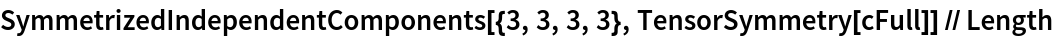Out=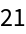One plane of symmetry, rotated by 180 degrees, results in the same stiffness:

 In:=Out=This results in a stiffness tensor with fewer independent components:

 In:=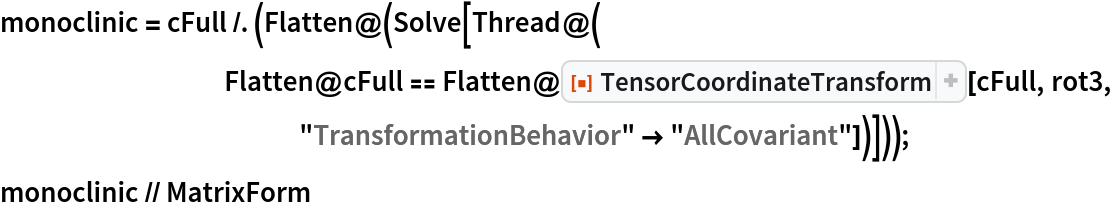Out=Determination and comparison of different material symmetries:

 In:=In:=In:=Summary:

 In:=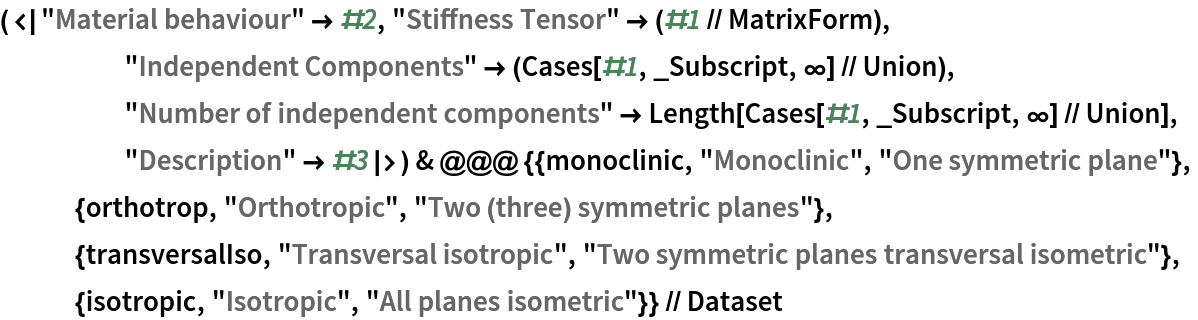Out=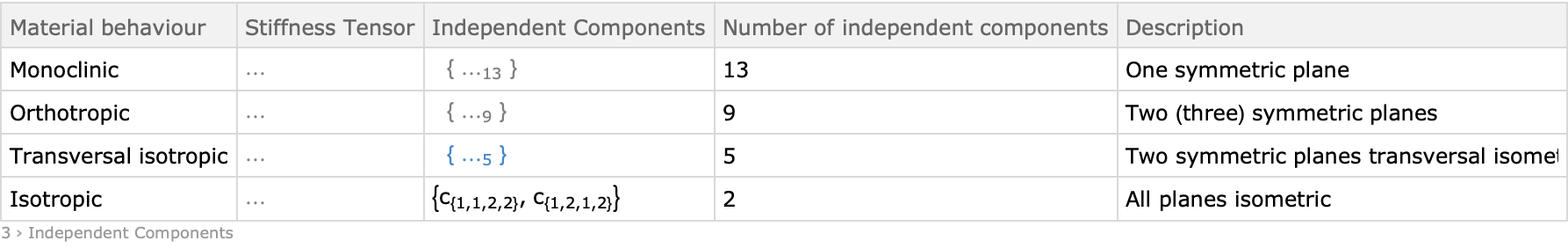Lars Ulke-Winter

## Version History

• 1.0.0 – 29 August 2019